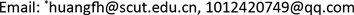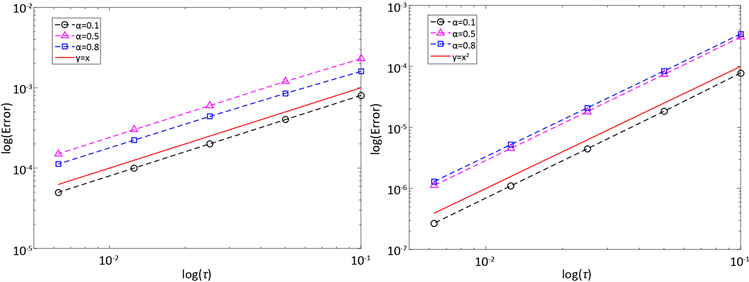﻿ 齐次分数阶扩散方程的加权C-N格式及其修正 Weighted C-N Scheme of HomogeneousFractional Di?usion Equations and Its Correction

Vol. 08  No. 10 ( 2019 ), Article ID: 32653 , 8 pages
10.12677/AAM.2019.810189

Weighted C-N Scheme of Homogeneous Fractional Diﬀusion Equations and Its Correction

Zhu Chen, Fenghui Huang*

Department of Mathematics, South China University of Technology, Guangzhou GuangdongReceived: Oct. 1st, 2019; accepted: Oct. 17th, 2019; published: Oct. 24th, 2019ABSTRACT

For the homogeneous time fractional diffusion equation, the accuracy of the numerical method will decrease when the exact solution is not smooth enough. In this case, we consider a weighted Crank-Nicolson scheme (masked as weighted C-N scheme) and its correction. After correcting the first step of the weighted C-N scheme, the second-order time accuracy can be restored. Then we give the detailed convergence analysis, and numerical examples verify the effectiveness of the scheme.

Keywords:Time Fractional Diﬀusion Equation, Crank-Nicolson Scheme, Convergence1. 引言

$\left\{\begin{array}{l}{}_{0}{}^{C}D{}_{t}{}^{\alpha }u\left(x,t\right)-Au\left(x,t\right)=0,\text{}L (1)

${}_{0}{}^{C}D{}_{t}{}^{\alpha }u\left(x,t\right)=\left\{\begin{array}{l}\frac{1}{\Gamma \left(1-\alpha \right)}{\int }_{0}^{t}{\left(t-s\right)}^{-\alpha }{u}^{\prime }\left(s\right)\text{d}s,\text{}0<\alpha <1\\ \frac{\text{d}u\left(x,t\right)}{\text{d}t},\text{}\alpha =1\end{array}$ (2)

$‖{\left(z-A\right)}^{-1}‖\le C{|z|}^{-1},\text{}\forall z\in {\Sigma }_{\omega }=\left\{z\ne 0:|\mathrm{arg}z|<\omega \right\},\text{}\omega \in \left(\frac{\pi }{2},\pi \right)$ (3)

2. 加权C-N格式及其修正格式

$V\left(t\right)=u\left(t\right)-{u}_{0}$，则方程(1)可表示为：

${}_{0}{}^{C}D{}_{t}{}^{\alpha }V\left(x,t\right)-AV\left(x,t\right)=A{u}_{0}$ (4)

$\tau$ 为时间步长， ${t}_{n}=n\tau ,\text{\hspace{0.17em}}n=0,1,2,\cdots ,N,\text{\hspace{0.17em}}0\le {t}_{n}\le T$，h为空间步长， ${x}_{i}=L+ih,\text{\hspace{0.17em}}i=0,1,\cdots ,M,\text{\hspace{0.17em}}L\le {x}_{i}\le R$${\stackrel{¯}{\partial }}_{t}^{\alpha }V\left({t}_{n}\right):={\tau }^{-\alpha }\underset{j=0}{\overset{n}{\sum }}{b}_{n-j}{V}^{j}$ 表示 ${}_{0}{}^{R}D{}_{t}{}^{\alpha }u\left(x,t\right)$ 的向后Euler卷积逼近，其生成函数为：

$\stackrel{˜}{b}\left(\xi \right)=\underset{j=0}{\overset{\infty }{\sum }}{b}_{j}{\xi }^{j}={\left(1-\xi \right)}^{\alpha }$ (5)

${\tau }^{-\alpha }\underset{j=0}{\overset{n}{\sum }}{b}_{n-j}{V}^{j}-\left(1-\frac{\alpha }{2}\right)A{V}^{n}-\frac{\alpha }{2}A{V}^{n-1}=A{u}_{0},\text{}n=1,2,\cdots ,N$ (6)

$\left\{\begin{array}{l}{\tau }^{-\alpha }\underset{j=0}{\overset{n}{\sum }}{b}_{n-j}{V}^{j}-\left(1-\frac{\alpha }{2}\right)A{V}^{n}-\frac{\alpha }{2}A{V}^{n-1}=\left(1+{c}_{0}\right)A{u}_{0},\text{}n=1\\ {\tau }^{-\alpha }\underset{j=0}{\overset{n}{\sum }}{b}_{n-j}{V}^{j}-\left(1-\frac{\alpha }{2}\right)A{V}^{n}-\frac{\alpha }{2}A{V}^{n-1}=A{u}_{0},\text{}n=2,\cdots ,N\end{array}$ (7)

3. 加权C-N修正格式的收敛性分析

$\mu \left(z\right)=\frac{1-{\text{e}}^{-\tau z}}{{\left(1-\frac{\alpha }{2}+\frac{\alpha }{2}{\text{e}}^{-\tau z}\right)}^{\frac{1}{\alpha }}}\cdot \frac{{c}_{0}{\text{e}}^{-\tau z}+\frac{{\text{e}}^{-\tau z}}{1-{\text{e}}^{-\tau z}}}{1-\frac{\alpha }{2}+\frac{\alpha }{2}{\text{e}}^{-\tau z}}$ (8)

$|\mu \left(z\right)-1|\le C{\tau }^{2}{|z|}^{2}$ (9)

$K\left(z\right)={z}^{-1}{\left({z}^{\alpha }-A\right)}^{-1}A$ (10)

${z}_{\tau }=\frac{1-{\text{e}}^{-\tau z}}{{\left(1-\frac{\alpha }{2}+\frac{\alpha }{2}{\text{e}}^{-\tau z}\right)}^{\frac{1}{\alpha }}}$ (11)

$‖K\left(z\right)‖\le C{|z|}^{-1}$ (12)

$‖K\left({z}_{\tau }\right)‖\le C{|z|}^{-1}$ (13)

$‖{\left({z}^{\alpha }-A\right)}^{-1}‖\le C{|z|}^{-\alpha }$ (14)

$‖{\left({z}_{\tau }^{\alpha }-A\right)}^{-1}‖\le C{|z|}^{-\alpha }$ (15)

${\left({z}^{\alpha }-A\right)}^{-1}A={z}^{\alpha }{\left({z}^{\alpha }-A\right)}^{-1}-I$ (16)

$‖K\left(z\right)‖={|z|}^{-1}‖{z}^{\alpha }{\left({z}^{\alpha }-A\right)}^{-1}-I‖\le C{|z|}^{-1}$ (17)

$‖K\left({z}_{\tau }\right)‖={|{z}_{\tau }|}^{-1}‖{z}_{\tau }^{\alpha }{\left({z}_{\tau }^{\alpha }-A\right)}^{-1}-I‖\le C{|z|}^{-1}$ (18)

$‖K\left({z}_{\tau }\right)-K\left(z\right)‖\le C{\tau }^{2}|z|$ (19)

$|{z}_{\tau }-z|\le C{\tau }^{2}{z}^{3}$ (20)

$|{z}_{\tau }^{\alpha }-{z}^{\alpha }|\le C{|z|}^{\alpha -1}|{z}_{\tau }-z|\le C{\tau }^{2}{|z|}^{2+\alpha }$ (  引理B.1)，且由式(16)及可得：

$\begin{array}{l}‖K\left({z}_{\tau }\right)-K\left(z\right)‖=‖{z}_{\tau }^{-1}{\left({z}_{\tau }^{\alpha }-A\right)}^{-1}A-{z}^{-1}{\left({z}^{\alpha }-A\right)}^{-1}A‖\\ \le {|{z}_{\tau }|}^{-1}‖{\left({z}_{\tau }^{\alpha }-A\right)}^{-1}A-{\left({z}^{\alpha }-A\right)}^{-1}A‖+|{z}_{\tau }^{-1}-{z}^{-1}|‖{\left({z}^{\alpha }-A\right)}^{-1}A‖\\ \le {|{z}_{\tau }|}^{-1}‖{z}_{\tau }^{\alpha }{\left({z}_{\tau }^{\alpha }-A\right)}^{-1}-{z}^{\alpha }{\left({z}^{\alpha }-A\right)}^{-1}‖+|{z}_{\tau }^{-1}-{z}^{-1}|‖{z}^{\alpha }{\left({z}^{\alpha }-A\right)}^{-1}-I‖\\ \le C{|z|}^{-1}|{z}_{\tau }^{\alpha }-{z}^{\alpha }|‖{\left({z}_{\tau }^{\alpha }-A\right)}^{-1}‖+C{|z|}^{-1}{|z|}^{\alpha }‖{\left({z}_{\tau }^{\alpha }-A\right)}^{-1}-{\left({z}^{\alpha }-A\right)}^{-1}‖+C\frac{|z-{z}_{\tau }|}{|z{z}_{\tau }|}\\ \le C{\tau }^{2}{|z|}^{-1}{|z|}^{2+\alpha }{|z|}^{-\alpha }+C{|z|}^{\alpha -1}‖\left({z}^{\alpha }-{z}_{\tau }^{\alpha }\right){\left({z}_{\tau }^{\alpha }-A\right)}^{-1}{\left({z}^{\alpha }-A\right)}^{-1}‖+C{\tau }^{2}|z|\le C{\tau }^{2}|z|\end{array}$ (21)

$\stackrel{^}{V}\left(z\right)={z}^{-1}{\left({z}^{\alpha }-A\right)}^{-1}A{u}_{0}$ (22)

$V\left(t\right)=\frac{1}{2\pi i}{\int }_{\Gamma }{\text{e}}^{tz}{z}^{-1}{\left({z}^{\alpha }-A\right)}^{-1}A{u}_{0}\text{d}z=\frac{1}{2\pi i}{\int }_{\Gamma }{\text{e}}^{tz}K\left(z\right){u}_{0}\text{d}z$ (23)

${\tau }^{-\alpha }\stackrel{˜}{b}\left(\xi \right)\stackrel{˜}{V}\left(\xi \right)-\left(1-\frac{\alpha }{2}+\frac{\alpha }{2}\xi \right)A\stackrel{˜}{V}\left(\xi \right)=\left({c}_{0}\xi +\frac{\xi }{1-\xi }\right)A{u}_{0}$ (24)

$\stackrel{˜}{V}\left(\xi \right)=\frac{{c}_{0}\xi +\frac{\xi }{1-\xi }}{1-\frac{\alpha }{2}+\frac{\alpha }{2}\xi }\cdot {\left[{\left(\frac{1-\xi }{\tau {\left(1-\frac{\alpha }{2}+\frac{\alpha }{2}\xi \right)}^{\frac{1}{\alpha }}}\right)}^{\alpha }-A\right]}^{-1}A{u}_{0}$ (25)

$\xi ={\text{e}}^{-\tau z}$，由Cauchy积分公式及式(8)、(10)和(11)可得加权C-N修正格式的数值解：

$\begin{array}{l}{V}^{n}=\frac{1}{2\pi i}{\int }_{|\xi |=\rho }{\xi }^{-n-1}\stackrel{˜}{V}\left(\xi \right)\text{d}\xi =\frac{\tau }{2\pi i}{\int }_{{\Gamma }_{\tau }}{\text{e}}^{{t}_{n}z}\stackrel{˜}{V}\left({\text{e}}^{-\tau z}\right)\text{d}z\\ \text{}=\frac{1}{2\pi i}{\int }_{{\Gamma }_{\tau }}{\text{e}}^{{t}_{n}z}\mu \left(z\right)K\left({z}_{\tau }\right){u}_{0}\text{d}z\end{array}$ (26)

$‖V\left({t}_{n}\right)-{V}^{n}‖\le C{\tau }^{2}{t}_{n}^{-2}‖{u}_{0}‖$ (27)

$‖\mu \left(z\right)K\left({z}_{\tau }\right)-K\left(z\right)‖\le C{\tau }^{2}|z|$ (28)

$\begin{array}{c}‖I‖\le \frac{1}{2\pi }{\int }_{{\Gamma }_{\tau }}|{\text{e}}^{{t}_{n}z}|‖\mu \left(z\right)K\left({z}_{\tau }\right)-K\left(z\right)‖\cdot ‖{u}_{0}‖\text{d}z\\ \le C{\int }_{{\Gamma }_{\tau }}|{\text{e}}^{{t}_{n}z}|{\tau }^{2}|z|‖{u}_{0}‖\text{d}z\\ \le C{\tau }^{2}{\int }_{0}^{\infty }{\text{e}}^{-cr}r{t}_{n}^{-1}{t}_{n}^{-1}‖{u}_{0}‖\text{d}r\\ \le C{\tau }^{2}{t}_{n}^{-2}‖{u}_{0}‖\end{array}$ (29)

$\begin{array}{c}‖I‖\le \frac{1}{2\pi }{\int }_{\Gamma /{\Gamma }_{\tau }}|{\text{e}}^{{t}_{n}z}|‖K\left(z\right)‖‖{u}_{0}‖\text{d}z\\ \le C{\int }_{\frac{1}{\tau }}^{\infty }{\text{e}}^{-c{t}_{n}|z|}{|z|}^{-2}|z|\text{d}z\\ \le C{\tau }^{2}{\int }_{0}^{\infty }{\text{e}}^{-cr}r{t}_{n}^{-1}{t}_{n}^{-1}‖{u}_{0}‖\text{d}r\\ \le C{\tau }^{2}{t}_{n}^{-2}‖{u}_{0}‖\end{array}$ (30)

4. 数值算例

$\left\{\begin{array}{l}{}_{0}{}^{C}D{}_{t}{}^{\alpha }u\left(x,t\right)-\frac{1}{4{\pi }^{2}}\frac{{\partial }^{2}u}{\partial {t}^{2}}=0,\text{}0 (31)Table 1. L 2 error of weighted C-N scheme (6) and weighted C-N modified scheme (7)Figure 1. The relationship between error and time step

$\left\{\begin{array}{l}{}_{0}{}^{C}D{}_{t}{}^{\alpha }u\left(x,t\right)-\frac{1}{4{\pi }^{2}}\frac{{\partial }^{2}u}{\partial {t}^{2}}=0,\text{}0 (32)Table 2. Error and time accuracy of weighted C-N scheme (6) and weighted C-N modified scheme (7)

Weighted C-N Scheme of HomogeneousFractional Di?usion Equations and Its Correction[J]. 应用数学进展, 2019, 08(10): 1611-1618. https://doi.org/10.12677/AAM.2019.810189

1. 1. Lubich, C., Sloan, I.H. and Thomee, V. (1996) Nonsmooth Data Error Estimates for Approximations of an Evolution Equation with a Positive-Type Memory Team. Mathematics of Computation, 65, 1-17.
https://doi.org/10.1090/S0025-5718-96-00677-1

2. 2. Lubich, C. (2004) Convolution Quadrature Revisited. Bit Numerical Mathematics, 44, 503-514.
https://doi.org/10.1023/B:BITN.0000046813.23911.2d

3. 3. Cuesta, E., Lubich, C. and Palencia, C. (2006) Convolution Quadrature Time Discretization of Fractional Diﬀusion-Wave Equations. Mathematics of Computation, 75, 673-696.
https://doi.org/10.1090/S0025-5718-06-01788-1

4. 4. Yan, Y.B., Khan, M. and Ford, N.J. (2018) An Analysis of the Modified L1 Scheme for Time-Fractional Partial Diﬀerential Equations with Nonsmooth Data. SIAM Journal on Numerical Analysis, 56, 210-227.
https://doi.org/10.1137/16M1094257

5. 5. Xing, Y.Y. and Yan, Y.B. (2018) A Higher Order Numerical Method for Time Fractional Partial Diﬀerential Equations with Nonsmooth Data. Journal of Computational Physics, 357, 305-323.
https://doi.org/10.1016/j.jcp.2017.12.035

6. 6. Tadjeran, C., Meerschaert, M.M. and Scheffler, H.P. (2006) A Second-Order Accurate Numerical Approximation for the Fractional Diﬀusion Equation. Journal of Computational Physics, 213, 205-213.
https://doi.org/10.1016/j.jcp.2005.08.008

7. 7. Jin, B.T., Lazarov, R. and Zhou, Z. (2018) An Analysis of the Crank-Nicolson Method for Subdiﬀusion. IMA Journal of Numerical Analysis, 38, 518-541.
https://doi.org/10.1093/imanum/drx019

8. 8. Jin, B.T., Lazarov, R. and Zhou, Z. (2017) Correction of High-Order BDF Convolution Quadrature for Fractional Evolution Equations. SIAM Journal on Scientific Computing, 39, A3129-A3152.
https://doi.org/10.1137/17M1118816